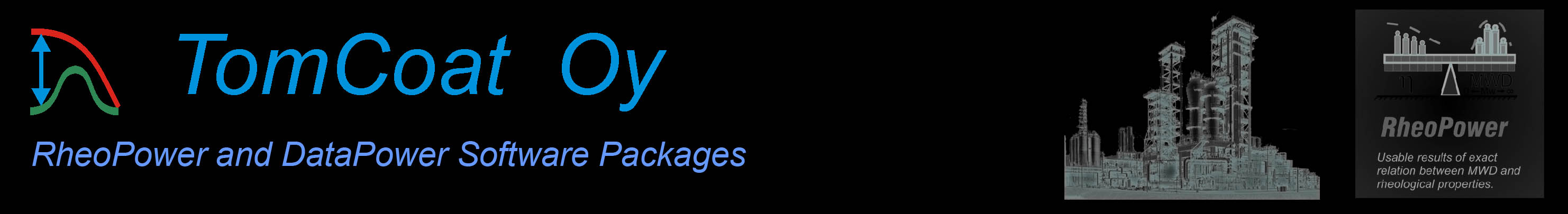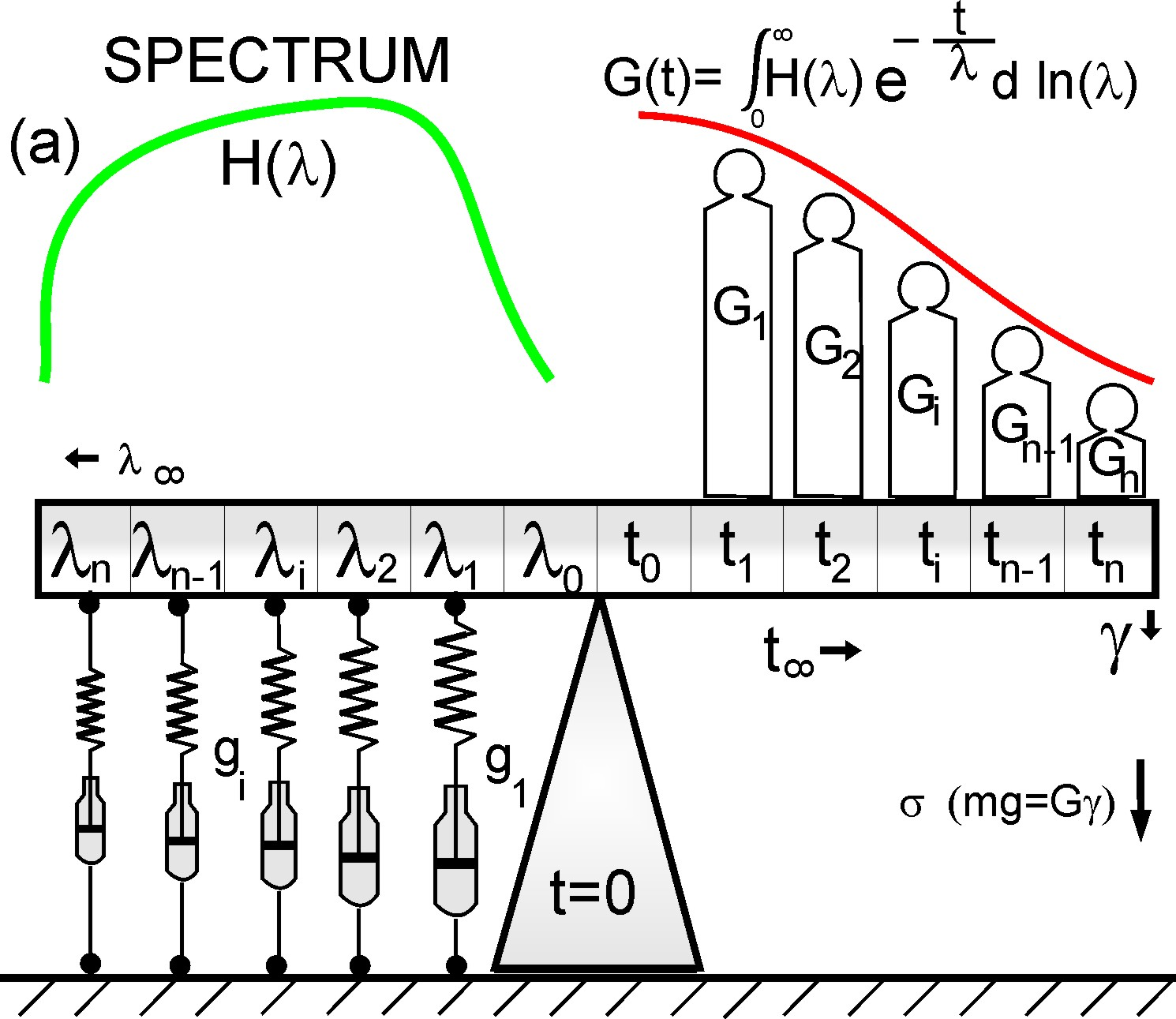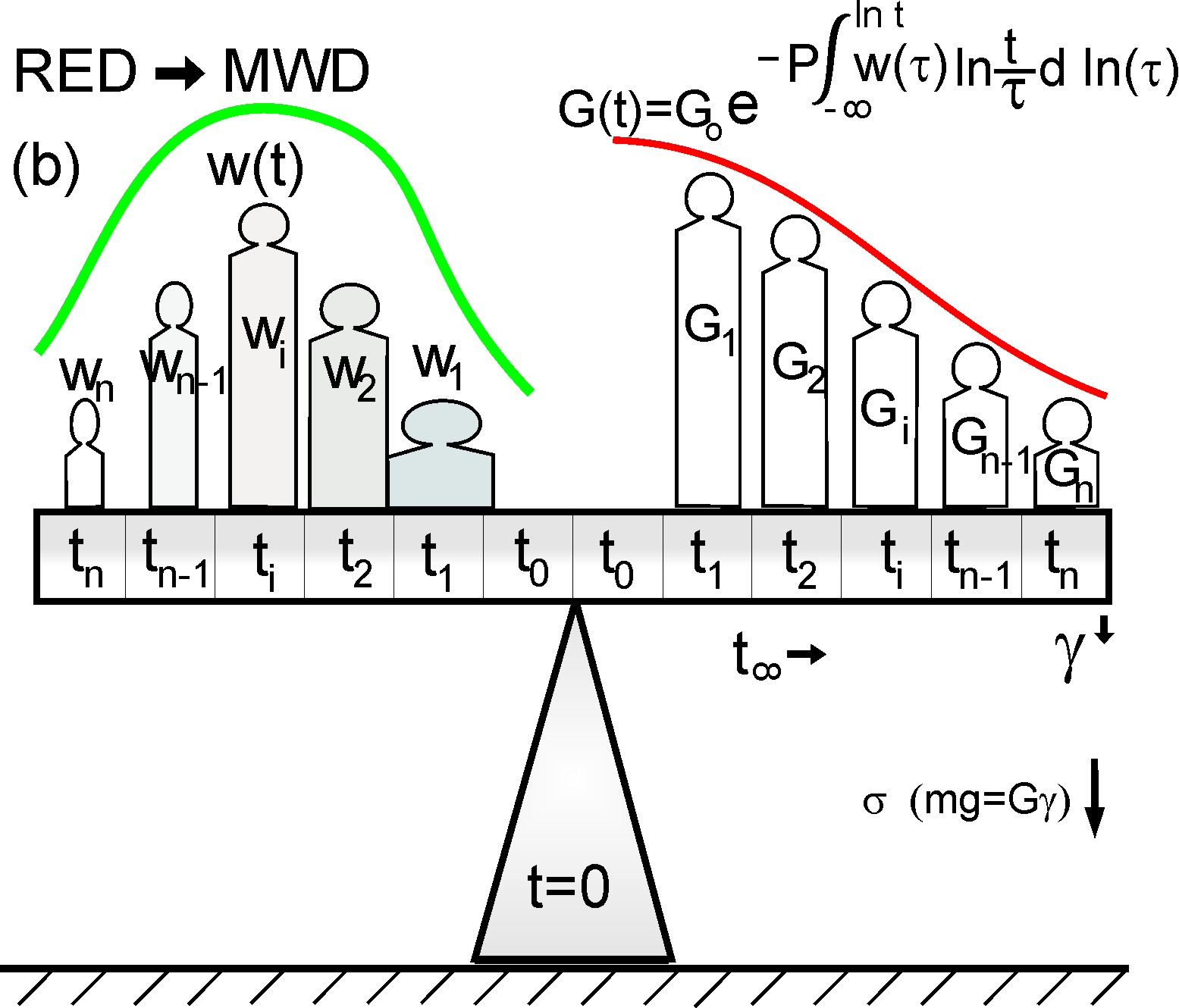Home
RheoPower >>
DataPower >>
News >>
FAQ >>
Wireframes
Hot Fix Archieve
SiteMap

Get RheoPower

XML Data Repository

## RheoPower Shortly by the Maxwell Model

Wireframe models.

Used model by RheoPower software package is described shortly by the help of well-known Maxwellian model. Some shortcomings and simplifies have to do as strictly speaking they do not relate each other. The aim is help reader to understand RheoPower model and introduce Control Theory to rheology.

### Building arrangement by Maxwell model

##### Schematic comparison of mechanical models by lever scales with dissimilar type integrals for relaxation modulus G(t) as a function of relaxation time λ or time t. The strongest element g1 or widest load w1 relates the longest and most effective chains. (a) Maxwell model of spring-dashpot arrangements for weight loads illustrating G(t).Maxwell model is normally described by a spring and dashpot arrangement. Generalized Maxwell model is illustrated by parallel arrangement of spring-dashpot elements and a stress force of relaxation modulus. We can illustrate the same by a lever scale, were on the left side are those spring-dashpot elements and respectively on the right side are relaxation moduli elements presented by loads in the wireframe. The loads on the bar are named as a function of time ti and respectively elements by relaxation time λi steps. As the lever is all the time in balance, we can neglect the distance to the turning point of the bar and model is linear in mathematical sense.

##### Nonlinear correction.In the case we add infinity amount of these spring-dashpot elements, we still cannot model the nonlinear behaviour of polymers. One solution could be additional some kind integrated circuit component (chip) illustrating correction function fi(t). In the arrangement is still needed some information for complicated non-linear corrections. This is an impossible task mechanically and even mathematically to get general procedure.

### Building arrangement for RheoPower model

##### (b) New model by Rheologically Effective Distribution (RED) relating directly MWD and the true structure of polymers.We have on the right side same relaxation modulus and on the left side are two Rheological Effective Distribution (RED) functions namely elastic RED' and viscous RED''. Weighted fractions wi are related to the MWD and polymer structure function P ∼ RED'+ RED''.

### Viscoelasticity

In Maxwell model springs depicts elasticity and dashpots viscous forces. Respectively in RheoPower Model RED describes the elasticity effects of flow and RED'' viscous and Rouse relaxation ranges, which influence with polymers mainly out of measurement range, but have still viscous effect on complete flow elsewhere.

### Mathematical treatment

In Maxwell model is summed the effects of all elements together giving us an integral equation. Respectively in RheoPower model relaxation impulse is summed by general Control Theory procedure. Despite the fact that RheoPower describes accurately relaxation phenomenon it can use for many other viscoelastic flows similar way.

Secondary on the both sides is used the same variable for the scale bar and mathematical formulas.

Finally RED is converted to Molecular Weight Distribution (MWD) by similar procedure as used with GPC/SEC, but here is used formula and method called Melt Calibration, which is now also linear procedure.
3928